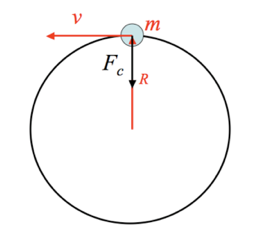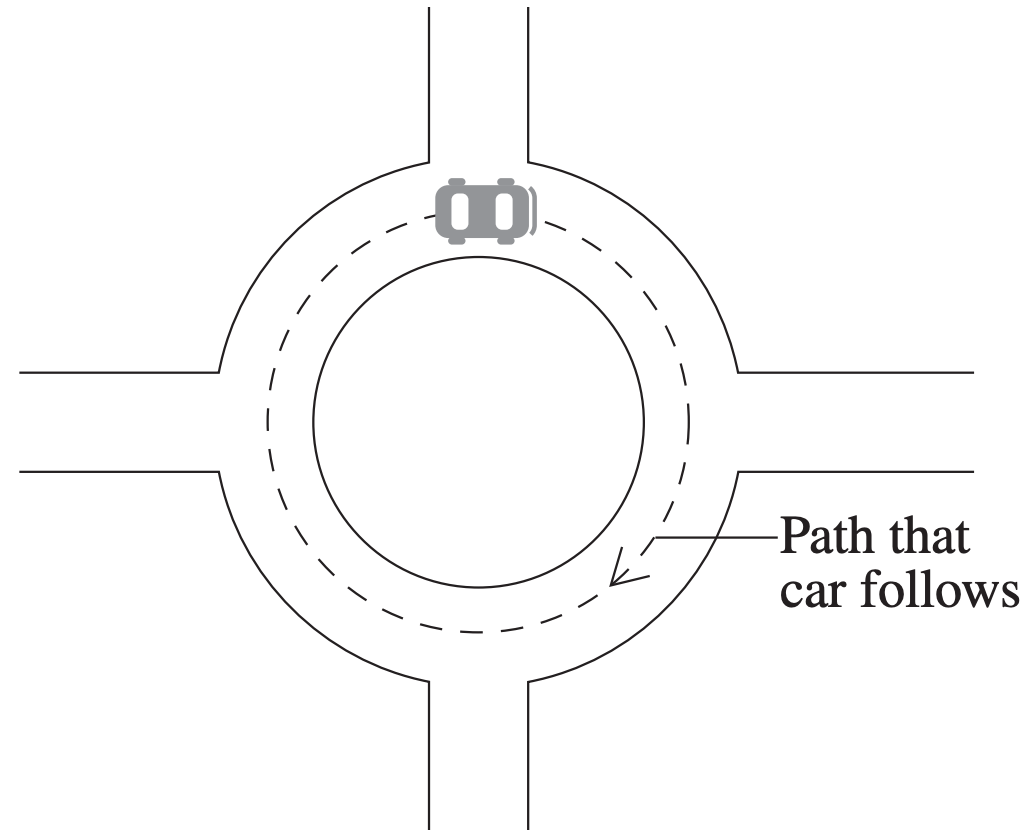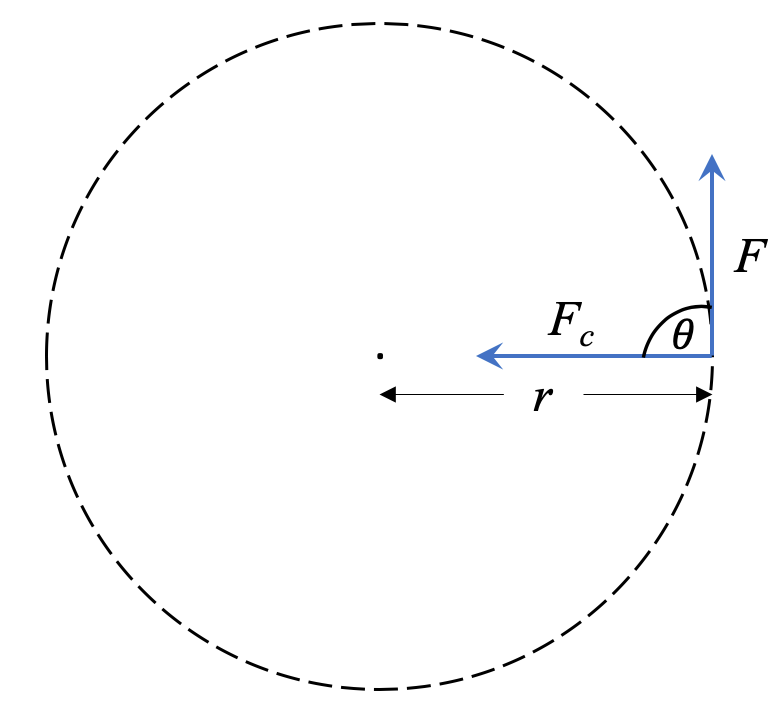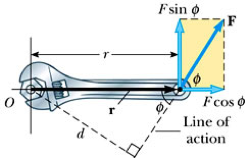# M5-S8: Torque & Work Done in Circular Motion

• ### Investigate the relationship between the total energy and work done on an object executing uniform circular motion:

- W=F_c*s*costheta

### Work done during uniform circular motion

During uniform circular motion, the speed of an object does not change which means its kinetic energy remains constant.

KE=1/2mv^2

This also means no work is done on the object as otherwise it would accelerate while gaining kinetic energy.While centripetal force acts on an object throughout uniform circular motion, it is not in the direction of its displacement so we cannot apply the formula W=Fd in this scenario.

The relationship between force and work done can be more accurately characterised by the following equation:

W=F_c*s*costheta

theta is the angle between direction of force and direction of displacement s of an object in circular motion.

In uniform circular motion, the net force (centripetal force) is acting orthogonal (90 degrees) to the object's direction of movement. This means costheta is always cos90º which equals zero.

Therefore, work done during uniform circular motion is zero.

## Practice Question 1 (HSC 2004)

A car with a mass of 800 kg travels at a constant speed of 7.5 m s−1 on a roundabout so that it follows a circular path with a radius of 16 m.A person observing this situation makes the following statement.

‘There is no net force acting on the car because the speed is constant and the friction between the tyres and the road balances the centripetal force acting on the car.’

Assess this statement. Support your answer with an analysis of the horizontal forces acting on the car, using the numerical data provided above.

• ### Investigate the relationship between the rotation of mechanical systems and the applied torque:

- tau=rFsintheta
- tau=r_(_|_)F

### Torque

In non-uniform circular motion, speed is not constant because it is acted on by force(s) other than centripetal force. When such a force is present, we have torque which is described by the equation:

tau=rFsintheta

r is the radius of circular motion or distance between the pivot and point of force contact. theta is the angle between the lever vector and applied force F that is causing the rotation/circular motion.When this angle is perpendicular, tau=rFsintheta becomes tau=r_(_|_)F.

Torque can be applied outside of circular motion in any situation involving turning about a pivot.Torque can be thought as the rotational analog of force. If force is a 'push or pull', then torque can be though as a twist. This is commonly applied in engineering e.g. using a wrench.The direction of torque is determined in the same way as angular velocity omega, by using the right-hand grip rule.

## Practice Question 2

How much torque is produced by opening a jar of pickles if the lid on the jar has a radius of 3.8 cm and the force exerted tangentially to the lid is 150 newtons?

## Practice Question 3

A 100 g pendulum swings in a clock, never swinging past an angle of 8 degrees with the vertical. If the pendulum swings on a 2.8-meter-long string attached to a pivot, what is the maximum amount of torque about the pivot?
Assuming there is negligible tension force in the rope.
If there is 0.5 N tension force in the rope.

## Practice Question 4

The model airplane has a mass of 0.90 kg and moves at constant speed on a circle that is parallel to the ground. The path of the airplane and the guideline lie in the same horizontal plane because the weight of the plane is balanced by the lift generated by its wings. Find the tension in the 17 m guideline for a speed of 19 m/s.